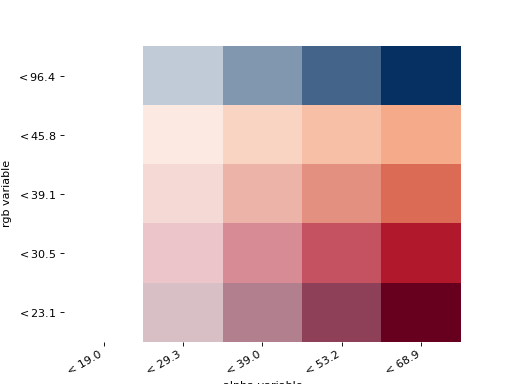splot.mapping.vba_legend¶

splot.mapping.vba_legend(rgb_bins, alpha_bins, cmap, ax=None)[source]

Creates Value by Alpha heatmap used as choropleth legend.

Parameters
rgb_binspysal.mapclassify instance

Object of classified values used for rgb. Can be created with mapclassify_bin() or pysal.mapclassify.

alpha_binspysal.mapclassify instance

Object of classified values used for alpha. Can be created with mapclassify_bin() or pysal.mapclassify.

axmatplotlib Axes instance, optional

Axes in which to plot the figure in multiple Axes layout. Default = None

Returns
figmatplotlip Figure instance

Figure of Value by Alpha heatmap

axmatplotlib Axes instance

Axes in which the figure is plotted

Examples

Imports

>>> from libpysal import examples
>>> import geopandas as gpd
>>> import matplotlib.pyplot as plt
>>> import matplotlib
>>> import numpy as np
>>> from splot.mapping import vba_legend, mapclassify_bin